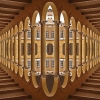#### You may also like### Not a Polite Question

When asked how old she was, the teacher replied: My age in years is not prime but odd and when reversed and added to my age you have a perfect square...### Whole Numbers Only

Can you work out how many of each kind of pencil this student bought?### Symmetricality

Add up all 5 equations given below. What do you notice? Solve the system and find the values of a, b, c , d and e. b + c + d + e = 4 a + c + d + e = 5 a + b + d + e = 1 a + b + c + e = 2 a + b + c + d = 0

# All Square

##### Age 11 to 14 Challenge Level:

Solve the system of equations

$\begin{eqnarray} \\ xy &=& 1 \\ yz &=& 4 \\ zx &=& 9. \end{eqnarray}$

Very good solutions came in from Christopher Kassam, age 13, Epsom; from Ian Green of Cooper's Coborn School; Lizzie and Sheli, Ruoyi Sun, Sarah Rogers, Arti Patel from the NLCS Puzzle Club; Alex Lam from St Peter's College, Adelaide; Beth Carroll, Sheila Luk, Alicia Maultby and Rachel Walker from the Mount School, York; Alex Filz from Ousedale School, Milton Keynes; Farhan Iskander, Foxford School, Coventry; and Christopher Dorrington and Lorn Tao, Stamford School. Well done all of you.

This is the solution from Lizzie and Sheli:
We realised that if you multiplied all the equations together and simplified it you ended up with:

$$x^2 y^2 z^2 =36$$

which, when square rooted, gives $xyz =\pm 6$. We know that $xy = 1$which means $z$ must equal $\pm 6$. We then worked out that as $xz = 9$, so $x \times\ \pm 6 = 9$, so $x$ must be $\pm 1.5$. Then $xy = 1$ , that is $\pm 1.5 \times\ y =1$ , so $y$ must be $\pm 0.6$ recurring (or two thirds).

The solutions are therefore

$x= 3/2$, $y= 2/3$ and $z = 6$ or $x=-3/2$, $y= -2/3$ and $z = -6$ (where $x, y$ and $z$ all have the same sign).